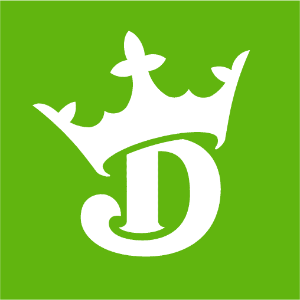DraftKings Super Bowl Odds
+600
6 to 1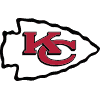Chiefs
11.7% implied probability

+650
6.5 to 1Ravens
10.9% implied probability

+900
9 to 1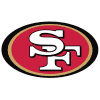49ers
8.2% implied probability

+1100
11 to 1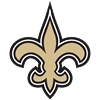Saints
6.8% implied probability

+1400
14 to 1Buccaneers
5.5% implied probability

+1600
16 to 1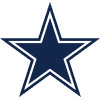Cowboys
4.8% implied probability

+2000
20 to 1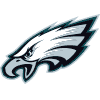Eagles
3.9% implied probability

+2200
22 to 1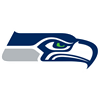Seahawks
3.6% implied probability

+2200
22 to 1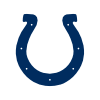Colts
3.6% implied probability

+2200
22 to 1Patriots
3.6% implied probability

+2500
25 to 1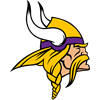Vikings
3.2% implied probability

+2500
25 to 1Steelers
3.2% implied probability

+2500
25 to 1Bills
3.2% implied probability

+2800
28 to 1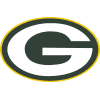Packers
2.8% implied probability

+2800
28 to 1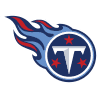Titans
2.8% implied probability

+4000
40 to 1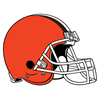Browns
2.0% implied probability

+4000
40 to 1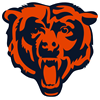Bears
2.0% implied probability

+4000
40 to 1Rams
2.0% implied probability

+4500
45 to 1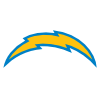Chargers
1.8% implied probability

+5000
50 to 1Cardinals
1.6% implied probability

+5000
50 to 1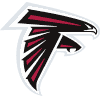Falcons
1.6% implied probability

+5000
50 to 1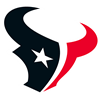Texans
1.6% implied probability

+5000
50 to 1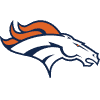Broncos
1.6% implied probability

+6000
60 to 1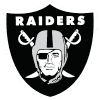Raiders
1.3% implied probability

+6600
66 to 1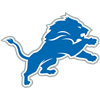Lions
1.2% implied probability

+8000
80 to 1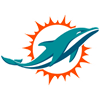Dolphins
1.0% implied probability

+8000
80 to 1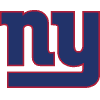Giants
1.0% implied probability

+8000
80 to 1Jets
1.0% implied probability

+10000
100 to 1Football Team
0.8% implied probability

No changes have been recorded yet.
+12500
125 to 1Panthers
0.7% implied probability

+15000
150 to 1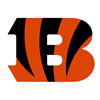Bengals
0.5% implied probability

+17500
175 to 1Jaguars
0.5% implied probability